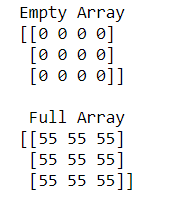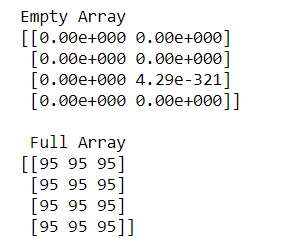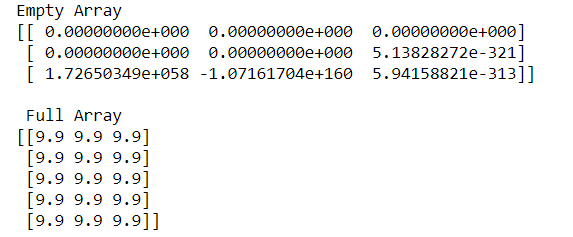GFG App
Open AppBrowser
Continue

# How to create an empty and a full NumPy array?

Sometimes there is a need to create an empty and full array simultaneously for a particular question. In this situation, we have two functions named as numpy.empty() and numpy.full() to create an empty and full arrays.

Syntax:

```numpy.full(shape, fill_value, dtype = None, order = ‘C’)
numpy.empty(shape, dtype = float, order = ‘C’)
```

Example 1:

## Python3

 `# python program to create ` `# Empty and Full Numpy arrays ` ` `  `import` `numpy as np ` ` `  ` `  `# Create an empty array ` `empa ``=` `np.empty((``3``, ``4``), dtype``=``int``) ` `print``(``"Empty Array"``) ` `print``(empa) ` ` `  `# Create a full array ` `flla ``=` `np.full([``3``, ``3``], ``55``, dtype``=``int``) ` `print``(``"\n Full Array"``) ` `print``(flla) `

Output:In the above example, we create an empty array of 3X4 and full array of 3X3 of INTEGER type.

Example 2:

## Python3

 `# python program to create ` `# Empty and Full Numpy arrays ` ` `  `import` `numpy as np ` ` `  ` `  `# Create an empty array ` `empa ``=` `np.empty([``4``, ``2``]) ` `print``(``"Empty Array"``) ` `print``(empa) ` ` `  `# Create a full array ` `flla ``=` `np.full([``4``, ``3``], ``95``) ` `print``(``"\n Full Array"``) ` `print``(flla) `

Output:In the above example, we create an empty array of 4X2 and full array of 4X3 of INTEGER and FLOAT type.

Example 3:

## Python3

 `# python program to create  ` `# Empty and Full Numpy arrays ` ` `  `import` `numpy as np ` ` `  `# Create an empty array ` `empa ``=`  `np.empty([``3``,``3``])  ` `print``(``"Empty Array"``) ` `print``(empa) ` ` `  `# Create a full array ` `flla ``=` `np.full([``5``,``3``], ``9.9``) ` `print``(``"\n Full Array"``) ` `print``(flla)`

Output:In the above example, we create an empty array of 3X3 and full array of 5X3 of FLOAT type.

My Personal Notes arrow_drop_up